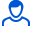Content: teorver_5589.doc (28.50 KB)

Positive responses: 0
Negative responses: 0

Refunds: 05589. x̅=20.2, n=16, σ=2. Find the confidence interval for estimating with a reliability of 0.95 the unknown mathematical expectation a of a normally distributed feature X of the general population, if the general standard deviation σ=2, the sample mean x̅v=20.2 and the sample size n=16 are known.
Detailed solution. Decorated in Microsoft Word 2003 (Quest decided to use the formula editor)
No feedback yet